Ask question

# Similar Triangles and Triginimetric Functions Use the figure below. Explain why /_ABC. /_ADE, and /_AFG are similar triangles.# Similar Triangles and Triginimetric Functions Use the figure below. Explain why /_ABC. /_ADE, and /_AFG are similar triangles.

Question
Similarityasked 2021-02-06
Similar Triangles and Triginimetric Functions Use the figure below.
Explain why $$\displaystyle\triangle{A}{B}{C}.\triangle{A}{D}{E},{\quad\text{and}\quad}\triangle{A}{F}{G}$$ are similar triangles.

## Answers (1)2021-02-07
Proof:
Consider the given triangle,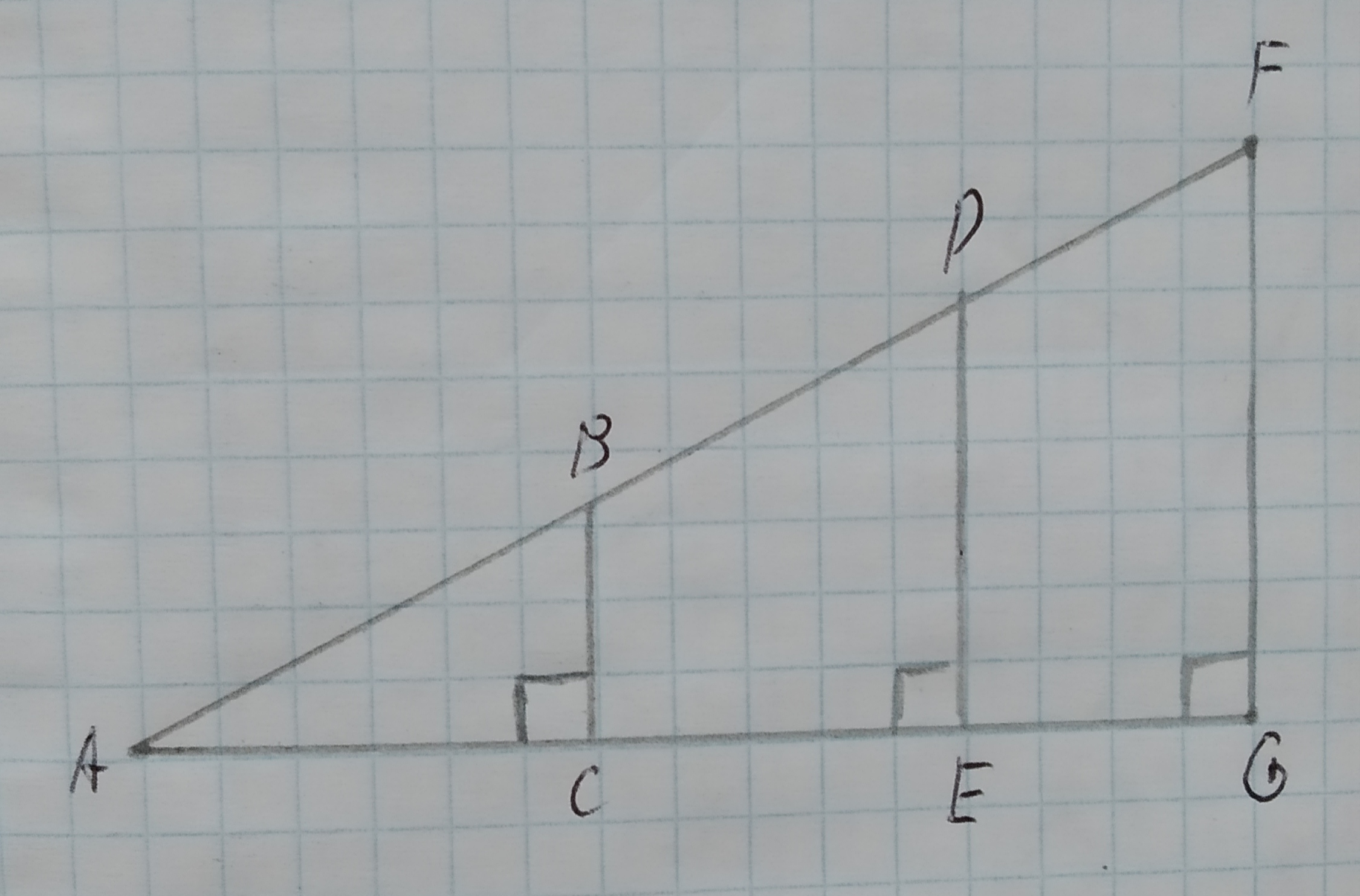The $$\displaystyle\angle{A}$$ is part of all three triangles $$\displaystyle\triangle{A}{B}{C},\triangle{A}{D}{E}{\quad\text{and}\quad}\triangle{A}{F}{G}$$ where, $$\displaystyle\angle{C}=\angle{E}=\angle{G}={90}^{{\circ}}$$.
Since , sum of all angles of a triangle is $$\displaystyle{180}^{{\circ}}$$ so from the triangle ABC,
$$\displaystyle\angle{A}+\angle{B}+\angle{C}={180}^{{\circ}}$$
$$\displaystyle\angle{B}={180}^{{\circ}}-\angle{A}-\angle{C}$$
$$\displaystyle={180}^{{\circ}}-\angle{A}-{90}^{{\circ}}$$
$$\displaystyle={90}^{{\circ}}-\angle{A}$$
In the triangle $$\displaystyle\triangle{A}{D}{E}$$,
$$\displaystyle\angle{A}+\angle{D}+\angle{E}={180}^{{\circ}}$$
$$\displaystyle\angle{D}={180}^{{\circ}}-\angle{A}-\angle{E}$$
$$\displaystyle={180}^{{\circ}}-\angle{A}-{90}^{{\circ}}$$
$$\displaystyle={90}^{{\circ}}-\angle{A}$$
Similarly, from triangle AFG the angle $$\displaystyle\angle{F}={90}^{{\circ}}—{Z}{A}$$.
Therefore, $$\displaystyle\angle{B}=\angle{D}=\angle{F}$$.
Since two of the angles of the triangles are equal, the third angle is also equal.
Therefore, the triangles are similar due to AAA similarity criterion.

### Relevant Questionsasked 2021-03-09
Similar Triangles and Triginimetric Functions Use the figure below.
What does similarity imply about the ratios $$\displaystyle\frac{{{B}{C}}}{{{A}{B}}},\frac{{{D}{E}}}{{{A}{D}}},{\quad\text{and}\quad}\frac{{{F}{G}}}{{{A}{F}}}$$?asked 2020-10-27
Given $$\displaystyle\triangle{D}{E}{F}{\quad\text{and}\quad}\triangle{E}{G}{F}$$ in the diagram below, determine if the triangles are similar. If so, write a similarity statement, and state the criterion used to support your claim.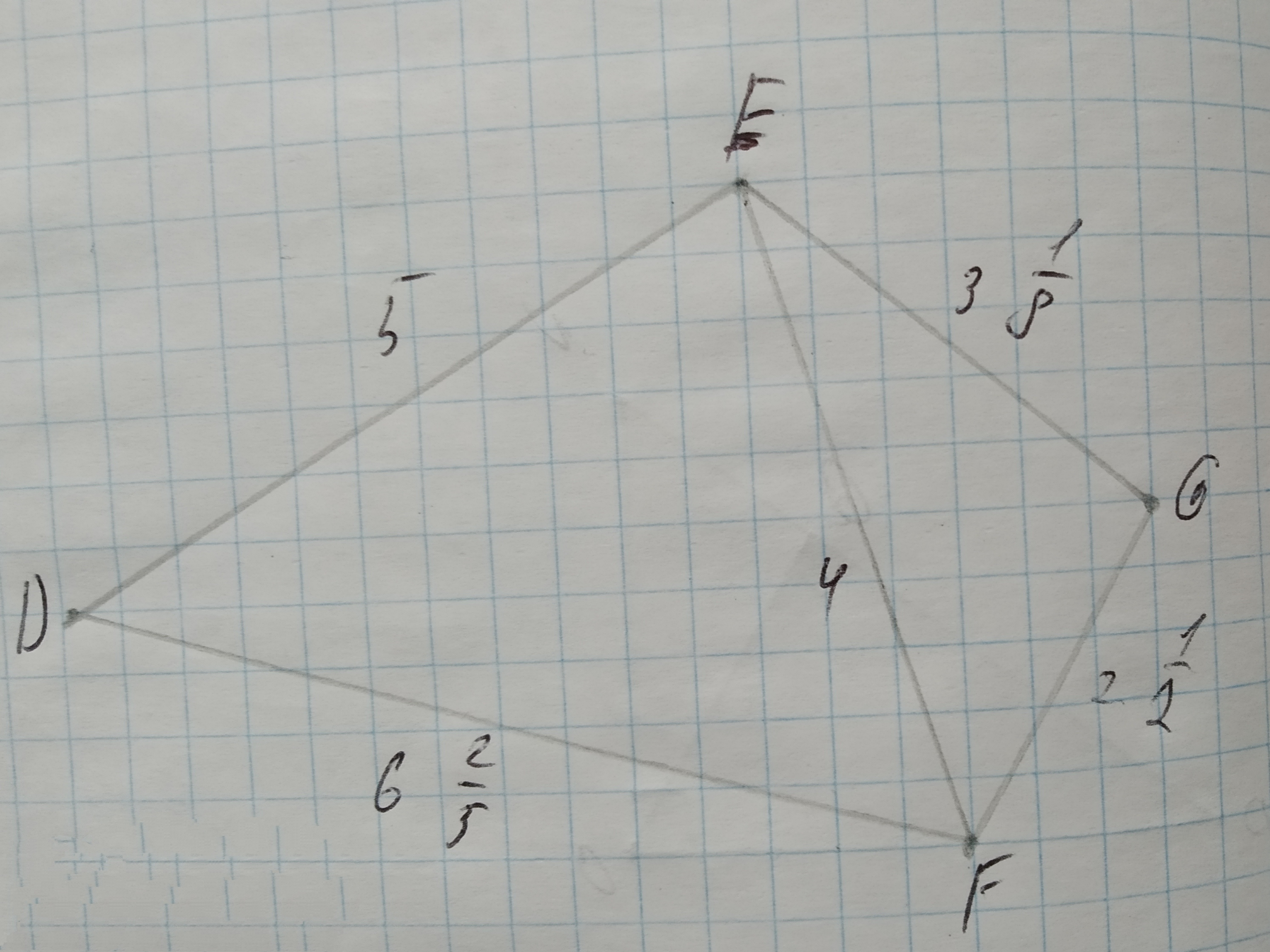asked 2021-01-07
To write three similarity statements about the triangles and the reason that they are similar.
Given that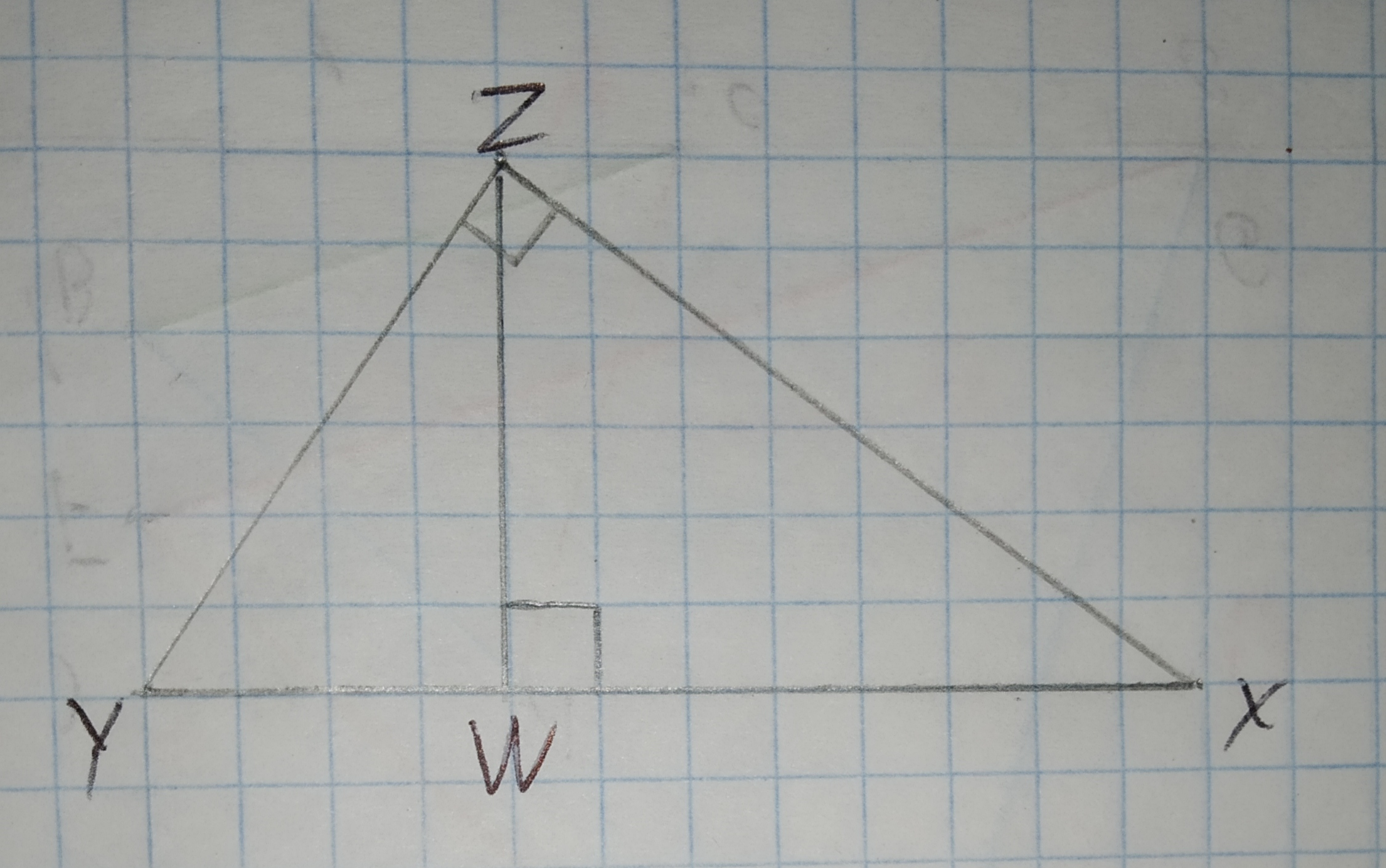asked 2020-10-27
To check: whether the triangles are similar. If so, write a similarity statement.
Given:
The given triangles are: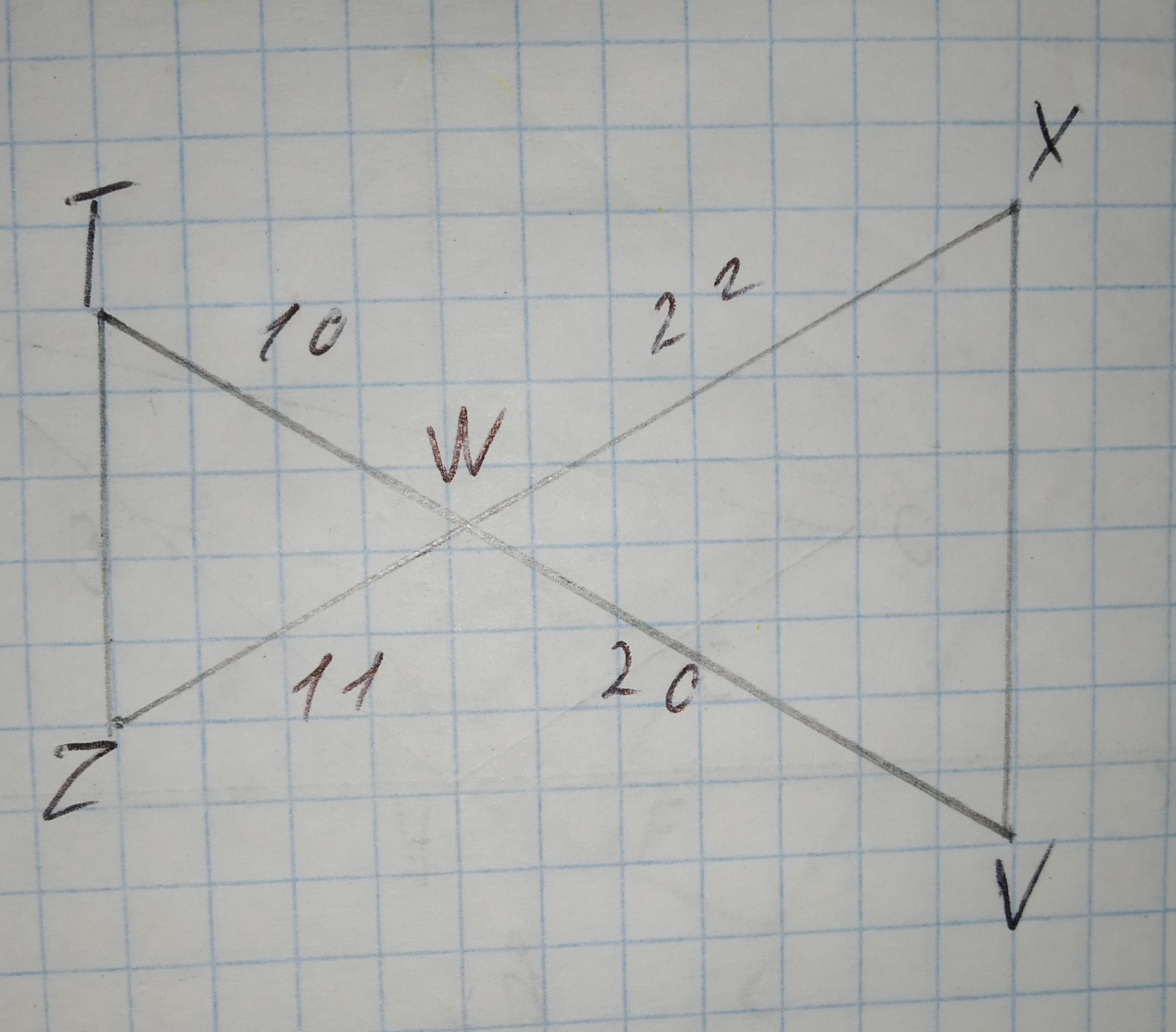asked 2020-11-30
To determine:To prove: $$\displaystyle\triangle{R}{S}{T}\sim\triangle{X}{Y}{Z}$$ by the SAS similarity theorem.
Given:
The triangles are similar by theorem SAS.
$$\displaystyle\angle{R}=\angle{X}$$ (Given)asked 2021-02-11
To determine:Given triangles are similar or not, if not give reason.
Given information: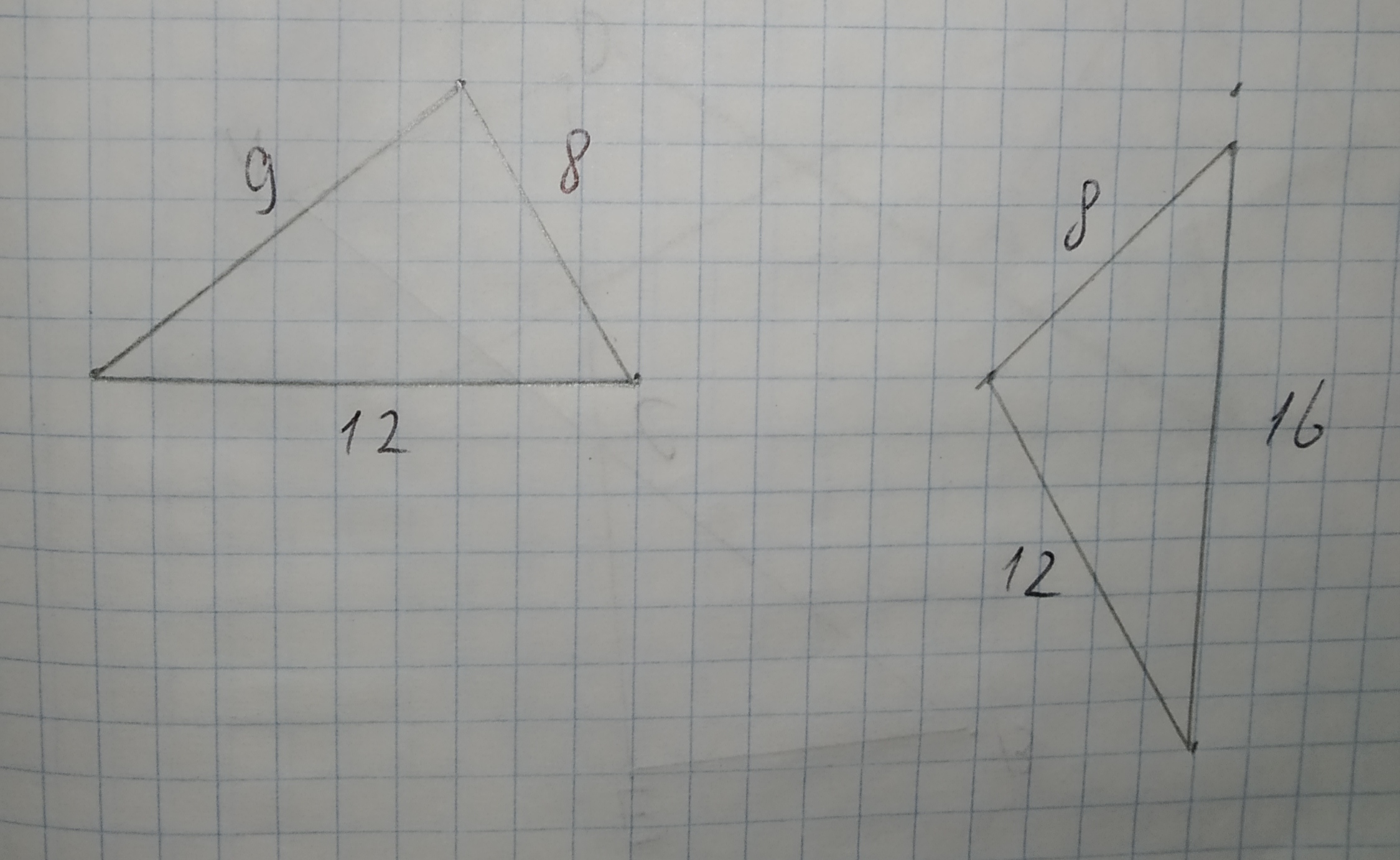asked 2020-10-27
Are pairs of triangles similar?
Given information: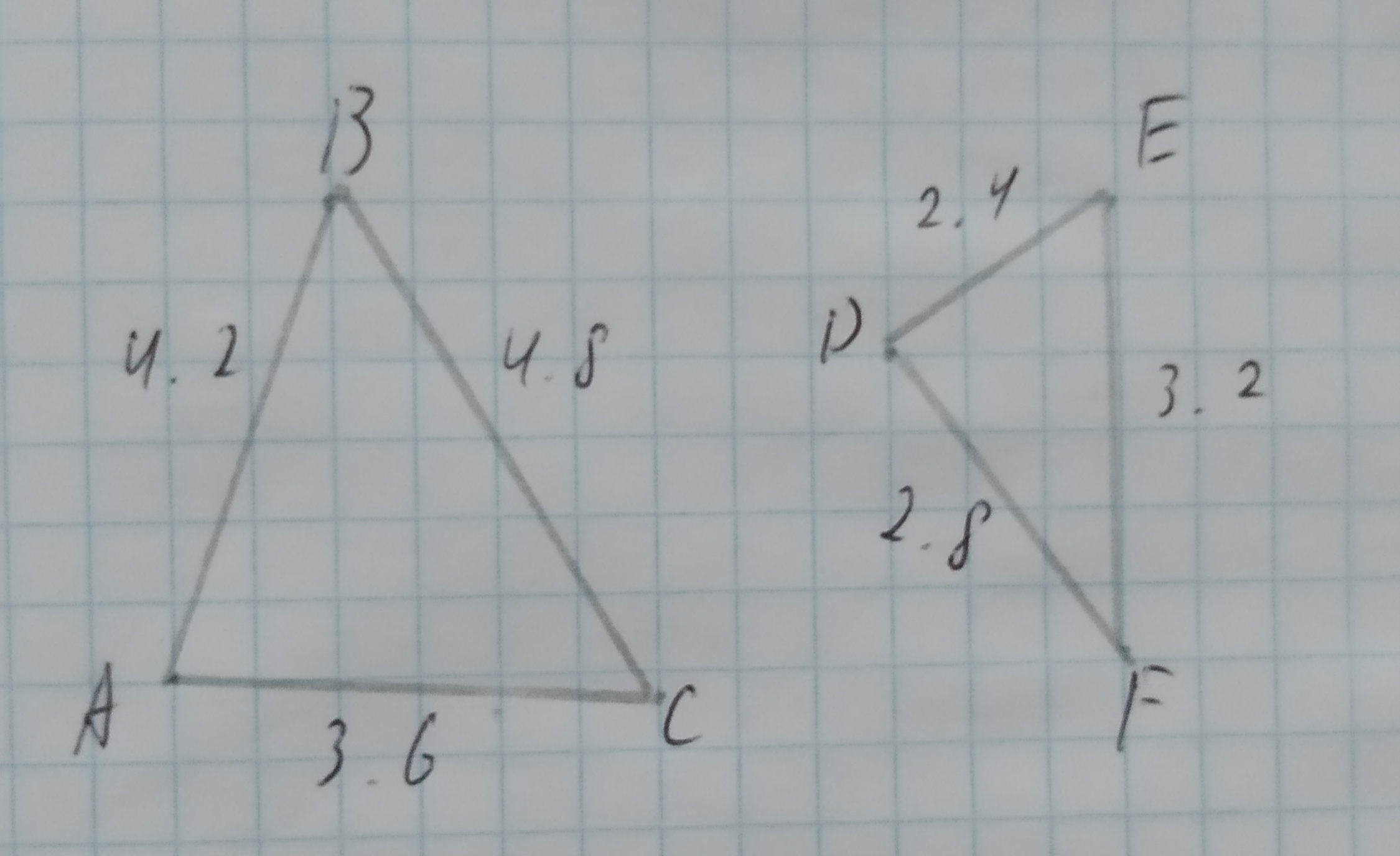asked 2021-01-31
Are pairs of triangles similar?
Given information: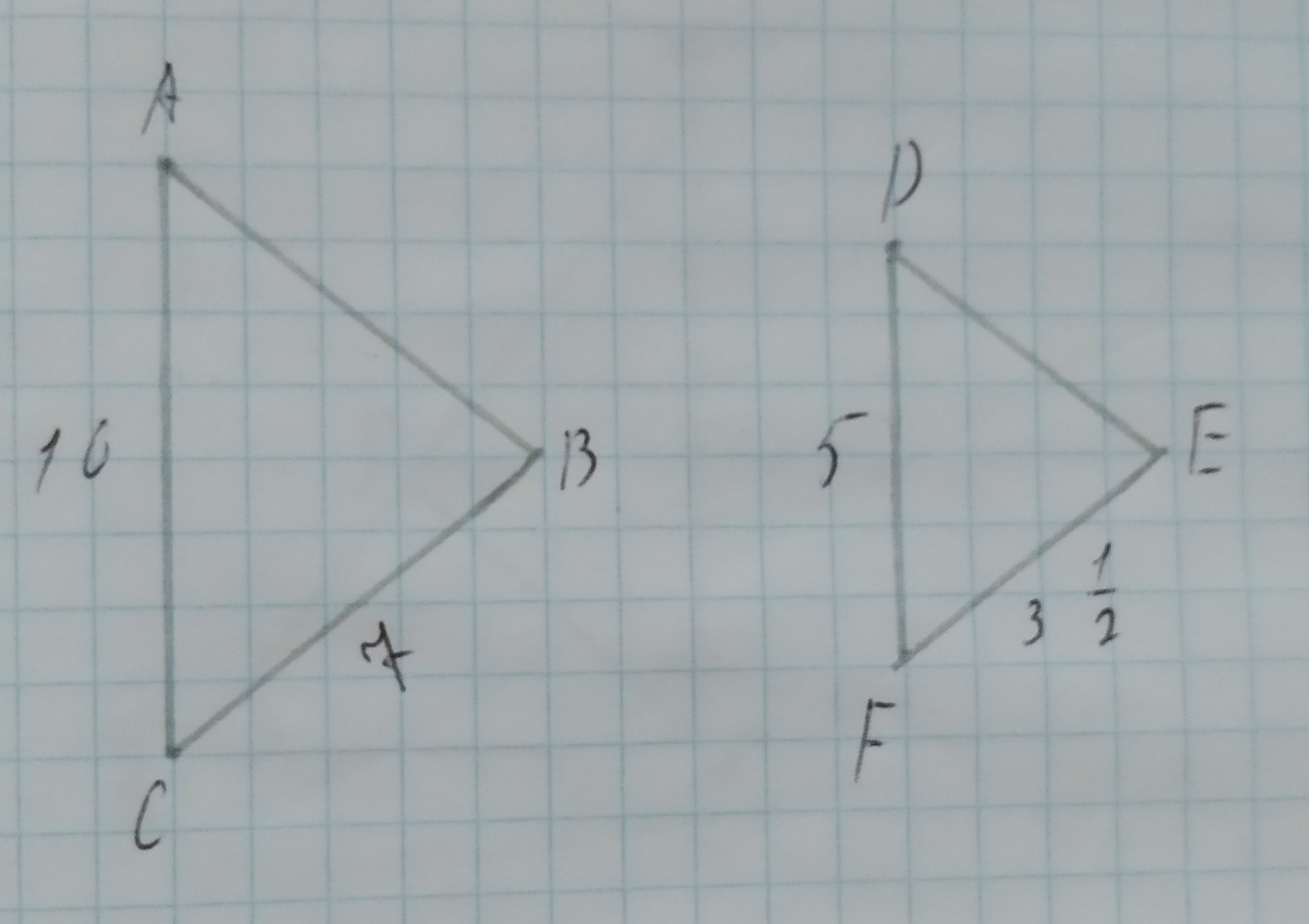asked 2021-02-26
Are pairs of triangles similar?
Given information: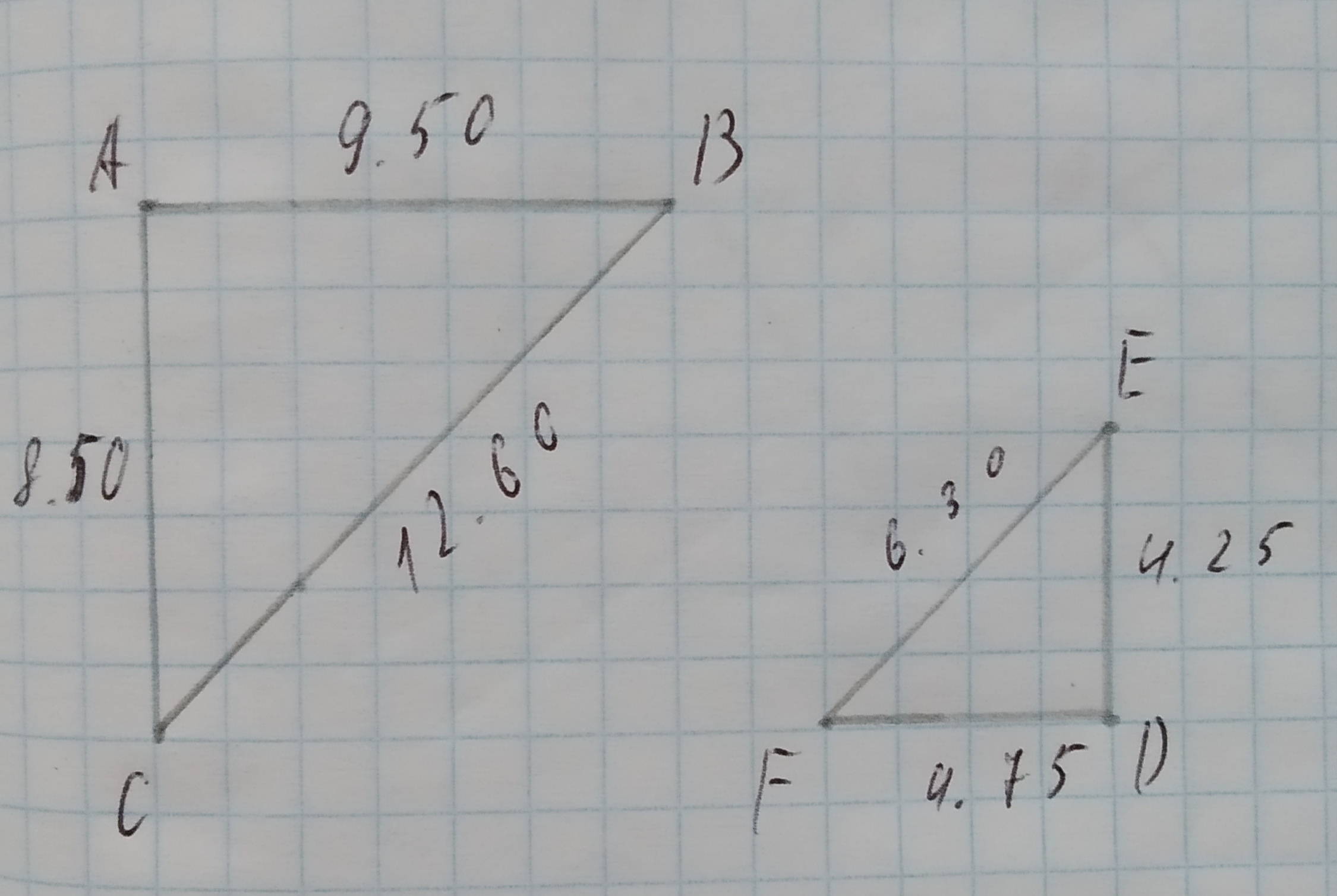asked 2021-02-06
Are pairs of triangles similar?
Given information: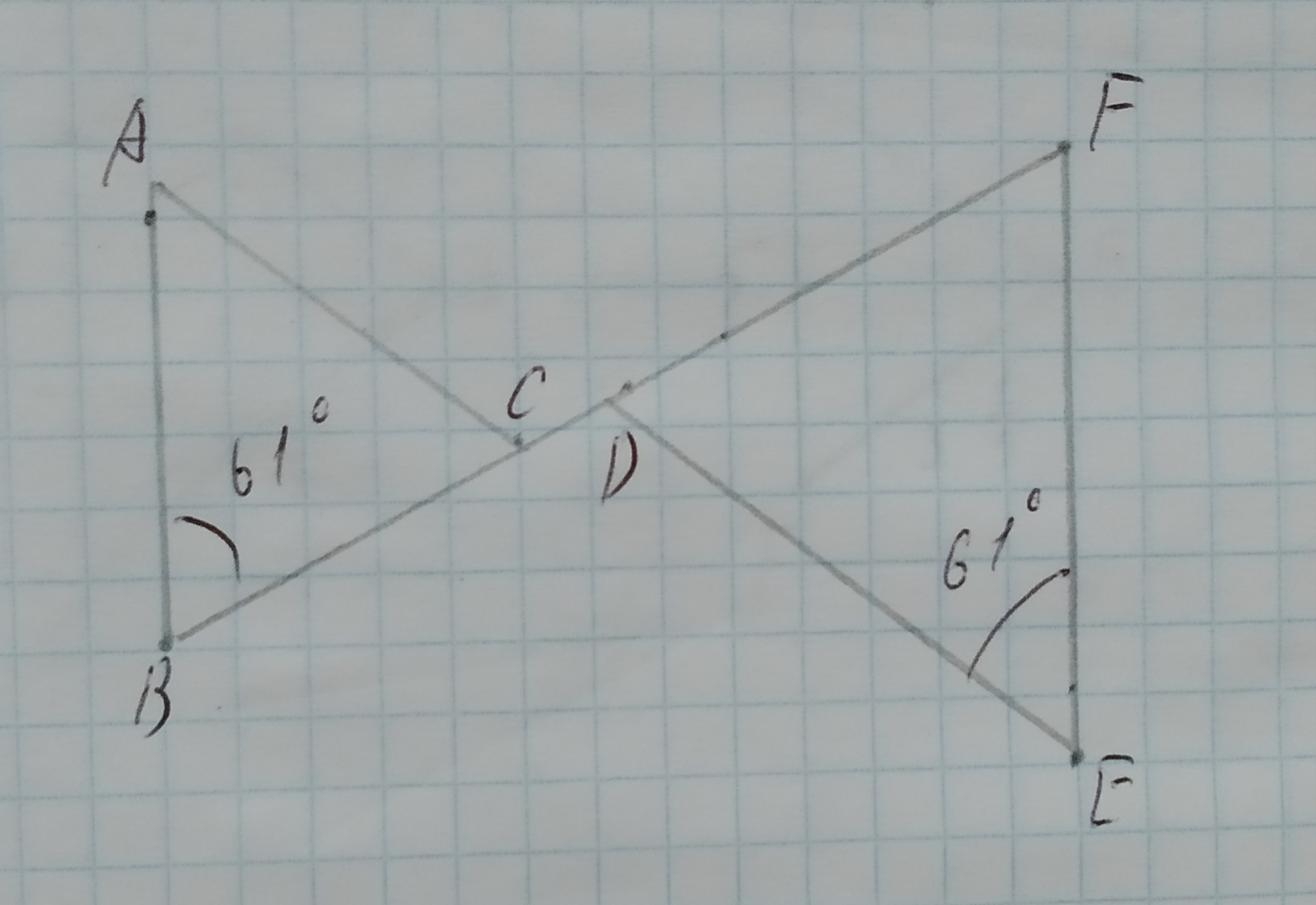...